SELF 76 S.B. Karavashkin and O.N. Karavashkina7.1.2. Check of pace of time in the mutually moving frames Now we will draw our attention to the technique with which bot Marder and Einstein derived the time intervals in the sequences of expressions (7.1)–(7.3) and (7.4)–(7.5). They both took some value of time at the given point of primed frame, these values are substituted to the right part of the most general form of Lorentz transform and then they found the moment of time in the second frame that relates to that chosen in the primed frame. In Marder’s derivation we can see the choice of a particular point in another frame only from the formulas (7.1) and (7.2), while Einstein wrote so: “Let in the coordinate origin of the frame K'  there is the clock with a second arm”. After it, the next value of time is taken at the same point of primed frame, and the same operation is done. Then the first value is subtracted from the second; so the time interval is yielded in the unprimed frame. And here the question arises: the time of which frame is compared with which time? Outwardly, this question seems to be rhetorical and if we transform in classical formalism, where the space-time transform is absent, this question would be senseless. But in the relativistic formalism this question has its grounds. If we use the Lorentz transforms in the cited way, we will see the known property of these transforms: to one moment of time in the primed frame there corresponds one value of coordinate in another (unprimed) frame, while to some other value of time in the primed frame there will correspond already other value of the coordinate in the unprimed frame. Actually, assume, one clock rests at the point (x', y', z') of the frame S' . Then at the moment T' , according to the Lorentz transforms, in the negative direction of motion of S (after Marder), (7.1) will be true. At the same time the following equality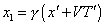(7.6)
 will be true. At the moment T' + 1  the equality (7.2) will be true, but the equality for another point of the frame S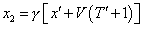(7.7)
 will be true, too. As we can see, comparing (7.6) and (7.7), we yield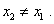(7.8)
 This evidences that according to the Einsteinian way to synch the time intervals, two clocks in the mutually moving frames cannot be checked. We can check only the clock of one frame with the physical time of another frame, as the transform equations cannot monitor another frame. They transform the parameters of body’s motion written relative to one frame into those written relative to another frame. This follows from the definition of frame and related properties of frame-to-frame transforms. Really, “to find the interlocation of the moving body (or point) with the body relative to which we study the motion, we strongly relate it to some coordinate system which in aggregate with the body forms the reference frame. If the coordinates of all points in the chosen frame remain constant, the body rests relative to this frame. If the coordinates of some points of a body change in time, the body relative to this frame (and, hence, relative to the body to which this frame relates) moves” [64, p. 138]. Thus, if the body A moved relative to the chosen frame, as it is shown in Fig. 7.1, we can state that namely this body moves relative to namely this frame. If we want to interrelate some accompanying frame with the body A, we transform the parameters of unprimed frame into that primed, as follows. First of all we find the equations of trajectory of motion of this body A in the initial frame.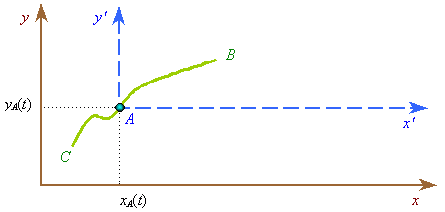Fig. 7.1. The motion of some body A in the initial reference frame xOy
 Suppose, the sought equations are given parametrically: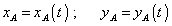(7.9)
 and the time inevitably is involved in these equations as the parameter, as the dynamic location of body in space can be described by the system of equations whose range is less than the number of variables; consequently, if speaking of the relativistic space-time, for the four dimensions we can compose only three equations of motion. The fourth equation will be excessive. Further, to build the reference frame related to the body A, we have to subtract the equations of motion of body A from the coordinates of primed frame. Then yield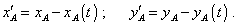(7.10)
 Since we have the system of equations of motion by one less than the number of variables, the fourth equation, i.e. the equation that usually describes the time transform, cannot be given in the transforms, basing on the equations of motion of the body to which we relate the moving frame. The same in case if some other body B moves relative to the body A. Then we yield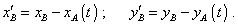(7.11)
 We see in the right part of (7.11) the characteristics of motion of bodies A and B from the point of frame which we took as resting, and both amounts of characteristics generally depend on time in this resting frame. In the left part we see the parameters of motion of the body B relative to the moving frame, i.e. to the body A. But in both descriptions we study the parameters of motion of one and the same body B. When the body moves along the axis x of the system xOy with the constant speed v, we have from (7.10)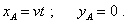(7.12)
 Relatively, (7.11) gains the form of known Galilee transform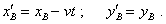(7.13)
 In the relativistic formalism, to these three (generally) transform equations we add also the equation of time transform, but it does not change the meaning of equations. They still will describe the trajectory of some body’s motion relative to two coordinate systems, and in the right part we see the parameters of body’s motion in the resting frame and characteristics of motion of the moving frame. Thus, if in the right parts of Marder’s and Einstein’s equations we see one and the same value of x’-component of a body related to the clock at different values of time, this means that we consider a body resting relative to the frame S' . Naturally, from the point of moving frame S this body has to move with the clock, just so we yield in (7.8) different values of the parameter x for different moments of time t' . Now, having cleared up the analysed feature of transforms, we can look at the Einsteinian statement “The clock moving with the speed v goes – from the point of non-accompanying coordinate system – slower than it would go should it rested”. Can the observer in the unprimed frame think the reading of clock that moves relative to his frame, – and just so it appears in the consideration of problem, – to be his time? No, of course, he could (and would have) to determine his time by the clock resting in his frame. To let him find it, we have to fix his clock in his frame. In this case we have to choose as constant the values of clock’s trajectory among the parameters in the right part of Lorentz transforms; then we will yield in the left the parameters of clock moving relative to the primed frame with the speed of unprimed frame. We will show it in more details after we have analysed, how much grounded it was – to introduce the time transform from the view of modelling of physical processes.

Contents: / 53 / 54 / 55 / 56 / 57 / 58 / 59 / 60 / 61 / 62 / 63 / 64 / 65 / 66 / 67 / 68 / 69 / 70 / 71 / 72 / 73 / 74 / 75 / 76 / 77 / 78 / 79 / 80 / 81 / 82 / 83 /